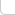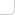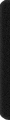# 某岛

… : "…アッカリ～ン . .. . " .. .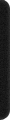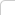July 25, 2015

# HDU 5306. Gorgeous Sequence

### Breif description:

There is a sequence a of length `n`. We use `ai` to denote the `i-th` element in this sequence. You should do the following three types of operations to this sequence.

• `0 x y t`: For every x≤i≤y, we use min(ai,t) to replace the original ai’s value.（区间取 min）
• `1 x y`: Print the maximum value of ai that x≤i≤y.（区间求最大値）
• `2 x y`: Print the sum of ai that x≤i≤y.（区间求和）

### Analysis:

• “>http://codeforces.com/contest/444/problem/C

• 暴力的线段树

```...
void upd(int x, int l, int r){  //上传信息
T[x].ss = T[lx].ss + T[rx].ss;
T[x].mx = max(T[lx].mx, T[rx].mx);
}

void pnc(int x, int l, int r, int t){ //打标记
if (T[x].mx <= t) return;

T[x].tag = t;

if (l < r){
pnc(lx, l, ml, t); pnc(rx, mr, r, t);
upd(xx);
}
else{
T[x].ss = T[x].mx = t;
}
}

void rls(int x, int l, int r){ //下放标记，因为我们是暴力打标记的。。所以暂时不需要。。
//pnc(lc, T[x].tag); pnc(rc, T[x].tag);
}
```

我们发现主要瓶颈是打标记这个步骤，因为在标记作用上的时刻，我们不知道它的子树中，有多少节点将会被这个标记影响。
因此需要递归到子树中去。。。

注意到这题中我们的 `tag` 标记是单调的，只需要留意子树中更小的标记。

```   4            4
2   6   ->   2   -
1 3 5 7      1 -
```

虽然这样第一行加了剪枝，然而并没有什么卵用。最坏情况下，递减的修改根节点，每次我们需要递归整棵树，复杂度 O(n)，和直接用数组暴力的复杂度一样。。。
。。。回忆 http://codeforces.com/contest/444/problem/C 。。。

我们注意到，在这种情况下，所有节点的 tag 信息总是相同的，我们理应利用这个信息，不要每次递归到整个子树中去。
而这要求我们多维护一个额外信息。。。

```struct rec{
LL ss; int mx, cv;
int tag;
} T[N];
```

这里 `cv` 表示，这个区间中有多少个结点，已被子树中的 `tag` 影响。

我们有：

```void upd(int x, int l, int r){
T[x].ss = T[lx].ss + T[rx].ss;
T[x].mx = max(T[lx].mx, T[rx].mx);
T[x].cv = T[lx].cv + T[rx].cv;
}

void pnc(int x, int l, int r, int t){
if (T[x].tag != Dummy && T[x].tag <= t) return;

T[x].tag = t;
if (T[x].cv != r-l+1){
T[x].mx = t;
T[x].ss += (LL) t * ((r-l+1)-T[x].cv);
T[x].cv = r-l+1;
}
}

void rls(int x, int l, int r){
if (T[x].tag != Dummy){
pnc(lc, T[x].tag); pnc(rc, T[x].tag);
}
}

```

。。。这样还是我们熟悉的普通线段树的写法。。。复杂度是对的。。但是问题在于，在打标记的时候，我们其实并不能直接维护 `cv`
因此在每次修改的时候，我们还需要添加一个 `fix` 函数，清理子树中所有大于等于 `t` 的标记。

```void Insert(int x, int l, int r){

if (T[x].mx <= t) return;

if (b < l || r < a) return;
if (a <= l && r <= b){
fix(xx, t);
if (l == r){
T[x].ss = T[x].mx = T[x].tag = t;
T[x].cv = 1;
}
else{
upd(xx);
}
pnc(xx, t);
}
else{
rls(xx);
Insert(lc); Insert(rc);
upd(xx);
}
}
```

。。。这样我们还剩 `fix` 函数需要考察。。。

```void fix(int x, int l, int r, int t){

if (T[x].mx < t) return;

if (T[x].tag >= t){
T[x].tag = Dummy;
}
if (l == r){
T[x].ss = T[x].mx = T[x].tag = T[x].tag;
T[x].cv = T[x].tag != Dummy;
}
else{
rls(xx);
fix(lc, t); fix(rc, t);
upd(xx);
}
}
```

。。。此时它已经和我们最初的方法有了很大的不同。。。。。

初看起来，每次 `Insert` 操作我们可能调用 `O(log(n))``fix` 函数，每次 `fix` 的复杂度又可能高达 `O(n)`

我们对其进行均摊分析！

当一个标记被清理成 `Dummy` 时，其子树中所有标记也都被赋成 `Dummy`，并且它的 `mx = 0`
我们考察最上面的那些 `Dummy` 标记，称之为 `封闭节点`
我们设置 `封闭节点``mx` 値等于 0，因而它们不会参与到 `fix` 函数的递归中去。

• 对于每次 `Insert` 操作，最多可能形成 `O(n)``封闭节点`
• 对于每次 `Insert` 操作，最多只会打开 `O(logn)``封闭节点`

因此总的时间复杂度仍为 `O(nlogn)`-> 完整代码